# Shnirel'man method

A method for adding sequences of positive integers; created by L.G. Shnirel'man in 1930. Letbe the number of elements of the sequence not larger than. Similarly to the measure of a set, one definesthe density of the sequence. A sequencethe elements of which are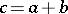,,, is called the sum of the two sequencesand.
Shnirel'man's theorem 1): If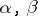are the densities of the summands, then the density of the sum is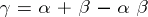. If after adding a sequence to itself a finite number of times one obtains the entire natural series, then the initial sequence is called a basis. In this case every natural number can be represented as the sum of a limited number of summands of the given sequence. A sequence with positive density is a basis.
Shnirel'man's theorem 2): The sequence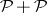has positive density, where the sequenceconsists of the number one and all prime numbers; hence,is a basis of the natural series, i.e. every natural numbercan be represented as the sum of a limited number of prime numbers. For the number of summands (Shnirel'man's absolute constant) the estimate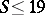has been obtained. In the case of representing a sufficiently large number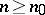by a sum of prime numbers with number of summands(Shnirel'man's constant), Shnirel'man's method together with analytical methods gives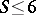. However, by the more powerful method of trigonometric sums of I.M. Vinogradov (cf. Trigonometric sums, method of) the estimate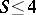was obtained.
Shnirel'man's method was applied to prove that the sequence consisting of the number one and of the numbers of the form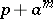, whereis a prime number,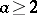is a natural number andis a basis of the natural series (N.P. Romanov, 1934).